#Fourier Series: Basic ResultsRecall that the mathematical expression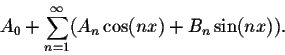is called a Fourier series.
Since this expression deals with convergence, we start by defining a similar expression when the sum is finite.

Definition. A Fourier polynomial is an expression of the form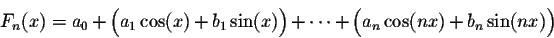which may rewritten as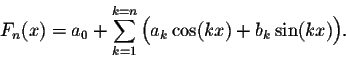The constants a0, ai and bi,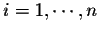, are called the coefficients of Fn(x).

The Fourier polynomials are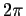-periodic functions. Using the trigonometric identities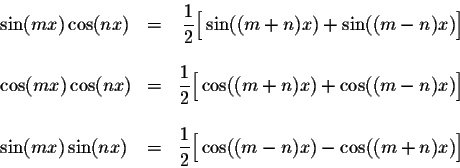we can easily prove the integral formulas
(1)
for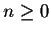, we have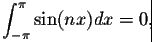for n>0 we have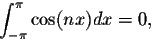(2)
for m and n, we have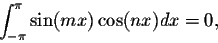(3)
for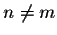, we have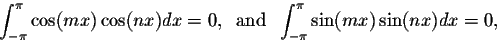(4)
for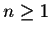, we have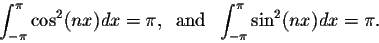Using the above formulas, we can easily deduce the following result:

Theorem. LetWe have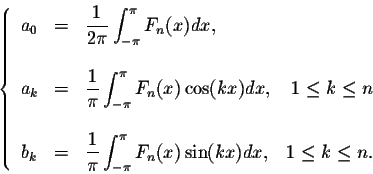This theorem helps associate a Fourier series to any-periodic function.

Definition. Let f(x) be a-periodic function which is integrable on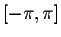. Set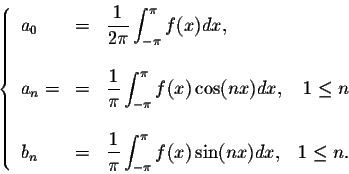The trigonometric series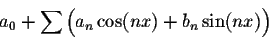is called the Fourier series associated to the function f(x). We will use the notation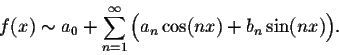Example. Find the Fourier series of the function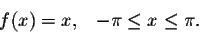Answer. Since f(x) is odd, then an = 0, for. We turn our attention to the coefficients bn. For any, we have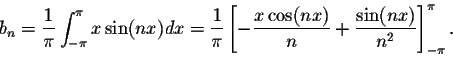We deduce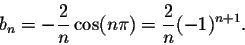Hence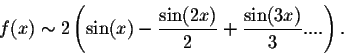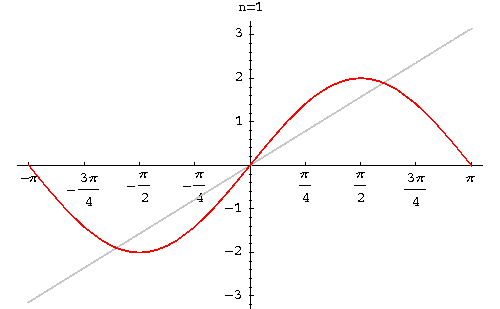Example. Find the Fourier series of the function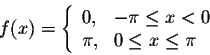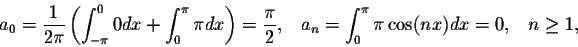and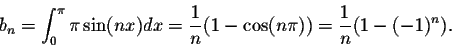We obtain b2n = 0 and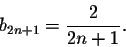Therefore, the Fourier series of f(x) is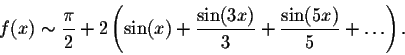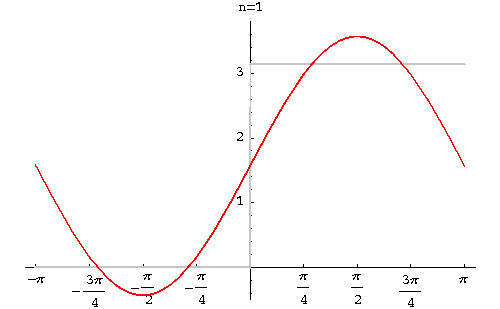Example. Find the Fourier series of the function function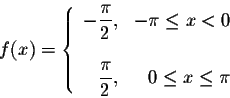Answer. Since this function is the function of the example above minus the constant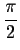. So Therefore, the Fourier series of f(x) is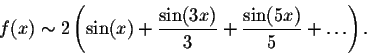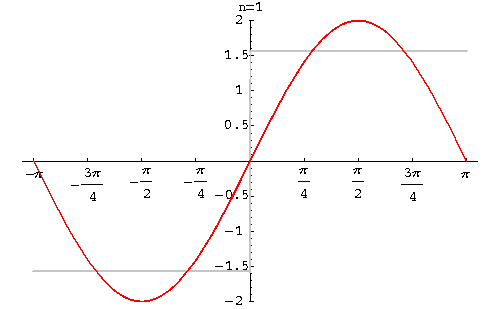Remark. We defined the Fourier series for functions which are-periodic, one would wonder how to define a similar notion for functions which are L-periodic.

Assume that f(x) is defined and integrable on the interval [-L,L]. Set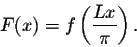The function F(x) is defined and integrable on. Consider the Fourier series of F(x)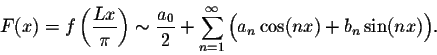Using the substitution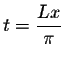, we obtain the following definition:

Definition. Let f(x) be a function defined and integrable on [-L,L]. The Fourier series of f(x) is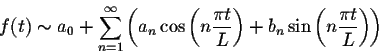where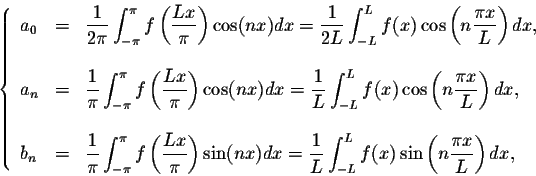for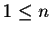.

Example. Find the Fourier series of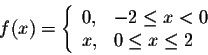Answer. Since L = 2, we obtain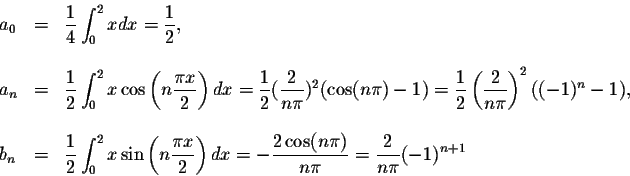for. Therefore, we have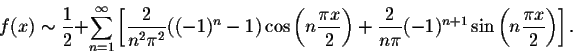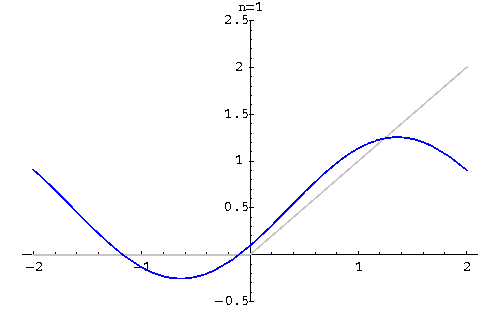[Geometry] [Algebra] [Trigonometry ]
[Calculus] [Differential Equations] [Matrix Algebra]S.O.S MATH: Home Page

Do you need more help? Please post your question on our S.O.S. Mathematics CyberBoard.Author: M.A. Khamsi